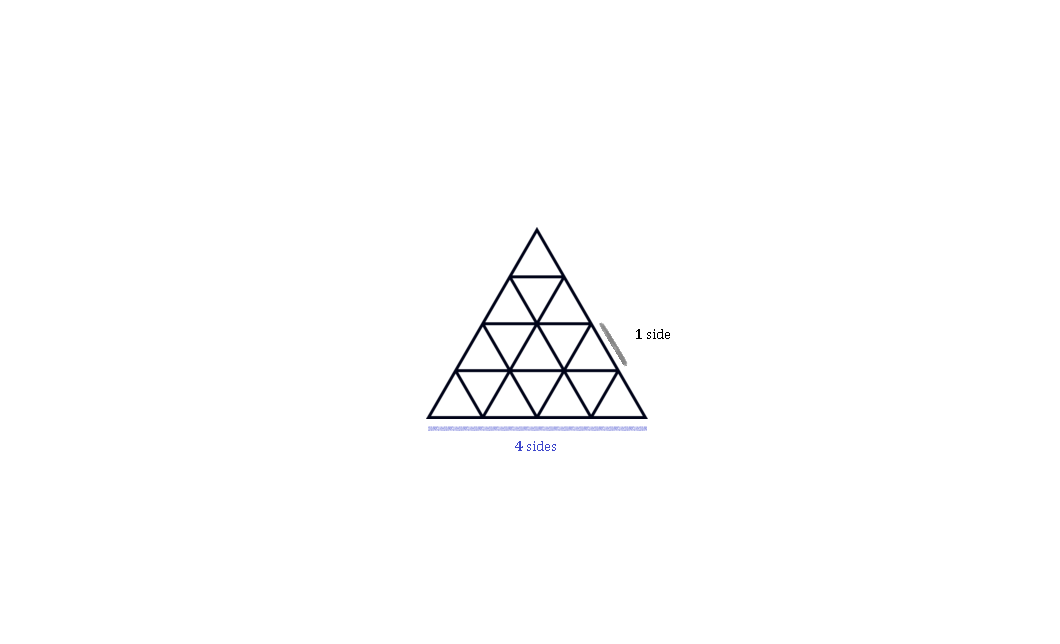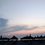# I will never have to (probably) count triangles ever again.

Note: This was done empirically, which means I have no solid proof for this shortcut. I am too lazy to figure out one but I'll leave it here for the sake of my hate for counting triangles. If anyone has a way to prove [or disprove] this, leave it on the comments.

Day: 10 Jan 2020, 23:55 (+01:00)

You are given to count the no. of triangles in an equaled cut triangle [you have to count more than just separated triangles]:This one has four sides. But I am too lazy to count. I figured a pattern that I wrote on this paper (someone LaTeX this):

Take a triangle made up of n sides, where 'n' is a Natural number. We are going to find the no. of spotted triangles where we do not need to count them. Take this list:

[1,3,4,6,7,9,10,12,...]

As you can see the pattern we use, this list in relation to number n, we need to take the first n digits of the list. If n = 7 ,we get: [1,3,4,6,7,9,10]

To find the number of triangles made up of n sides, we multiply each digits with ascending order by adding 1, starting with no. '1' starting to the left side of the list. So this would be (again, for n = 7 [it does not matter what 'n' is if it is a Natural no.]) :

(1 x 7) + (3 x 6) + (4 x 5) + (6 x 4) + (7 x 3) + (9 x 2) + (10 x 1)

where this equals 118.

A triangle with 7 sides has $118$ triangles in total. You can check that on Google if it is correct (or count).

I am sorry guys for the bad formatting and being so lazy to decorate it (and explaining why does this algorithm work so). I had a very bad day today and I do not feel like doing much. So yeah, "The proof is left as an exercise to the reader".Note by Nikolas Кraj
1 year, 5 months ago

This discussion board is a place to discuss our Daily Challenges and the math and science related to those challenges. Explanations are more than just a solution — they should explain the steps and thinking strategies that you used to obtain the solution. Comments should further the discussion of math and science.

When posting on Brilliant:

• Use the emojis to react to an explanation, whether you're congratulating a job well done , or just really confused .
• Ask specific questions about the challenge or the steps in somebody's explanation. Well-posed questions can add a lot to the discussion, but posting "I don't understand!" doesn't help anyone.
• Try to contribute something new to the discussion, whether it is an extension, generalization or other idea related to the challenge.
• Stay on topic — we're all here to learn more about math and science, not to hear about your favorite get-rich-quick scheme or current world events.

MarkdownAppears as
*italics* or _italics_ italics
**bold** or __bold__ bold
- bulleted- list
• bulleted
• list
1. numbered2. list
1. numbered
2. list
Note: you must add a full line of space before and after lists for them to show up correctly
paragraph 1paragraph 2

paragraph 1

paragraph 2

[example link](https://brilliant.org)example link
> This is a quote
This is a quote
    # I indented these lines
# 4 spaces, and now they show
# up as a code block.

print "hello world"
# I indented these lines
# 4 spaces, and now they show
# up as a code block.

print "hello world"
MathAppears as
Remember to wrap math in $$ ... $$ or $ ... $ to ensure proper formatting.
2 \times 3 $2 \times 3$
2^{34} $2^{34}$
a_{i-1} $a_{i-1}$
\frac{2}{3} $\frac{2}{3}$
\sqrt{2} $\sqrt{2}$
\sum_{i=1}^3 $\sum_{i=1}^3$
\sin \theta $\sin \theta$
\boxed{123} $\boxed{123}$

## Comments

There are no comments in this discussion.

×

Problem Loading...

Note Loading...

Set Loading...